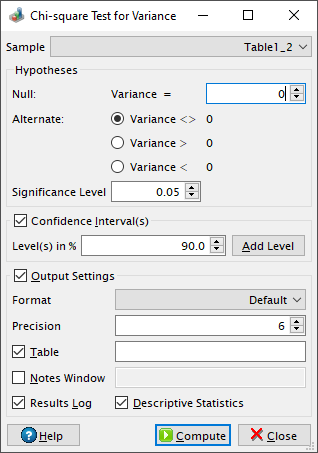# Chi-square Test Dialog

This dialog is activated by selecting the Chi-square Test for Variance... command from the Statistics -> Hypothesis Testing -> menu. A chi-squared test is any statistical hypothesis test where the sampling distribution of the test statistic is a chi-squared distribution when the null hypothesis is true. Please visit the Wikipedia article on the Chi-squared test for more details about this statistical test.

Figure 5-115. The Chi-square Test for Variance dialog.The chi-square compares the variance (V) of a population to a Vtest value specified in the null hypothesis. By default QtiPlot performs a two-tailed test, the default alternate hypothesis being that the variance of the population and the Vtest values are different (V <> Vtest). It is also possible to perform an upper-tailed test by choosing the alternate hypothesis that V > Vtest or a lower-tailed test (V < Vtest).

This test uses the Chi-Square statistic, χ2 = (N-1)V/Vtest, where N is the size of the population.

The test statistic is used to compute a probability (P value). For a lower-tailed test the probability is calculated using the formula p = chi2cdf(χ2, DoF), where the chi2cdf function calculates the lower tail of the cumulative distribution function for the chi-squared distribution with DoF = N-1 degrees of freedom. For an upper-tailed test, the probability is calculated using the formula p = 1-chi2cdf(χ2, DoF). For a two-tailed test the probability is calculated using the formulas p = chi2cdf(χ2, DoF) if chi2cdf(χ2, DoF) < 0.5 and p = 2[1-chi2cdf(χ2, DoF)] otherwise.

The null hypothesis is rejected if the calculated probability is lower than the value of the Significance Level.

If the Confidence Interval(s) box is checked QtiPlot also computes a confidence interval (Lower Limit and Upper Limit) for each user defined level.